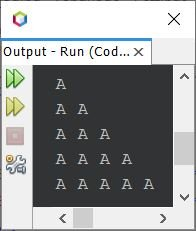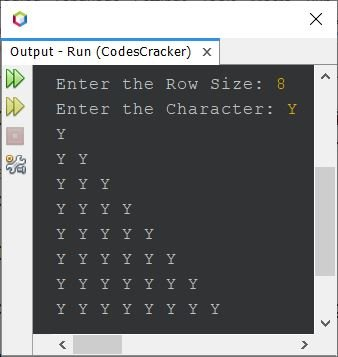# Java Program to Print Pattern of Alphabet Character

This article covers those programs in Java that prints the pattern of alphabet character. All the famous pattern program that can be created using alphabets, are covered here.

## Alphabet Pattern in Java - Pattern No.1

The question is, write a Java program to print pattern of alphabet. The answer to this question is the program given below. This program prints right-angled triangle of alphabet.

```public class CodesCracker
{
public static void main(String[] args)
{
int i, j, row=5;
char ch='A';

for(i=0; i<row; i++)
{
for(j=0; j<=i; j++)
System.out.print(ch+ " ");
System.out.print("\n");
}
}
}```

The snapshot given below shows the sample output produced by above program in Java on printing of alphabet pattern:To allow user to define the size of triangle, or the row size of pattern, use the program given below. This program also receives the character to form the pattern with:

```import java.util.Scanner;

public class CodesCracker
{
public static void main(String[] args)
{
int i, j, row;
char ch;
Scanner s = new Scanner(System.in);

System.out.print("Enter the Row Size: ");
row = s.nextInt();
System.out.print("Enter the Character: ");
ch = s.next().charAt(0);

for(i=0; i<row; i++)
{
for(j=0; j<=i; j++)
System.out.print(ch+ " ");
System.out.print("\n");
}
}
}```

The sample run of above program with user input 8 as row size and Y as character to form the pattern, is:That is, instead of manually or directly initializing the value of row and ch, initialization done using user input.

You can also try with a character, other than an alphabet, such as #, @, -, ., /, } etc.

## Alphabet Pattern in Java - Pattern No.2

The above program can also be created in a way, to print the next character each time, while forming the pattern:

```public class CodesCracker
{
public static void main(String[] args)
{
int row=5;
char ch='A';

for(int i=0; i<row; i++)
{
for(int j=0; j<=i; j++)
{
System.out.print(ch+ " ");
ch++;
}
System.out.print("\n");
}
}
}```

```A
B C
D E F
G H I J
K L M N O ```

## Alphabet Pattern in Java - Pattern No.3

Also the program can be created in a way that, each row starts with 'A' and rest of the things are similar to previous program. That is, A at first row, A B at second row, A B C at third row, and so on.

```public class CodesCracker
{
public static void main(String[] args)
{
int row=5;

for(int i=0; i<row; i++)
{
char ch='A';
for(int j=0; j<=i; j++)
{
System.out.print(ch+ " ");
ch++;
}
System.out.print("\n");
}
}
}```

```A
A B
A B C
A B C D
A B C D E ```

## Alphabet Pattern in Java - Pattern No.4

The program still can be modified to print the same right-angled triangle pattern of alphabet character, where the alphabets are in different form. That is, increment the alphabet at each row, but do not increment for columns. That is, A at first row, B B at second row, C C C at third row, and so on.

```public class CodesCracker
{
public static void main(String[] args)
{
int row=5;
char ch='A';

for(int i=0; i<row; i++)
{
for(int j=0; j<=i; j++)
System.out.print(ch+ " ");

System.out.print("\n");
ch++;
}
}
}```

```A
B B
C C C
D D D D
E E E E E ```

## Alphabet Pattern in Java - Pattern No.5

Now let me change the orientation of the pattern, to understand the different way of creating the pattern of alphabet character in Java.

```public class CodesCracker
{
public static void main(String[] args)
{
int row=5, sLimit;
sLimit = (row*2) - 2;
char ch = 'A';

for(int i=0; i<row; i++)
{
for(int space=0; space<sLimit; space++)
System.out.print(" ");
for(int j=0; j<=i; j++)
System.out.print(ch+ " ");
System.out.print("\n");
sLimit = sLimit-2;
}
}
}```

#### Output of Previous Alphabet Pattern Program

```        A
A A
A A A
A A A A
A A A A A ```

Note - You can try the same as done in previous programs to print the same shape with different looks of alphabet pattern.

## Alphabet Pattern in Java - Pattern No.6

```public class CodesCracker
{
public static void main(String[] args)
{
int row=5;
char ch='A';

for(int i=0; i<row; i++)
{
for(int j=i; j<row; j++)
System.out.print(ch+ " ");

System.out.print("\n");
}
}
}```

#### Output of Previous Alphabet Pattern Program

```A A A A A
A A A A
A A A
A A
A ```

## Alphabet Pattern in Java - Pattern No.7

```public class CodesCracker
{
public static void main(String[] args)
{
int row=5, sLimit=0;
char ch='A';

for(int i=0; i<row; i++)
{
for(int space=0; space<sLimit; space++)
System.out.print(" ");

for(int j=i; j<row; j++)
System.out.print(ch+ " ");

System.out.print("\n");
sLimit += 2;
}
}
}```

#### Output of Previous Alphabet Pattern Program

```A A A A A
A A A A
A A A
A A
A```

## Alphabet Pattern in Java - Pattern No.8

The pattern created using this program, looks like an equilateral triangle of alphabet.

```public class CodesCracker
{
public static void main(String[] args)
{
int row=5;
char ch='A';

for(int i=0; i<row; i++)
{
for(int space=i; space<row; space++)
System.out.print(" ");

for(int j=0; j<=i; j++)
System.out.print(ch+ " ");

System.out.print("\n");
}
}
}```

#### Output of Previous Alphabet Pattern Program

```     A
A A
A A A
A A A A
A A A A A ```

## Alphabet Pattern in Java - Pattern No.9

```public class CodesCracker
{
public static void main(String[] args)
{
int row=5;
char ch='A';

for(int i=0; i<row; i++)
{
for(int space=0; space<i; space++)
System.out.print(" ");

for(int j=i; j<row; j++)
System.out.print(ch+ " ");

System.out.print("\n");
}
}
}```

#### Output of Previous Alphabet Pattern Program

```A A A A A
A A A A
A A A
A A
A ```

Note - For more pattern, refer to Star Pattern Program in Java. The only change, you need to do, is to change the star with alphabet.

Java Online Test

« Previous Program Next Program »